# Computing and Computational Analysis

This online computing and computational analysis course is based an introductory computing course I gave at the School of Physics, University College Dublin, and on material in the book Do The Math! On Growth, Greed, and Strategic Thinking (Sage, 2013). Instruction, code ideas, exercises, and sample programs are given to get started writing programs for scientific and statistical analysis, using a number of instructional and popular languages. Let me know how you get on.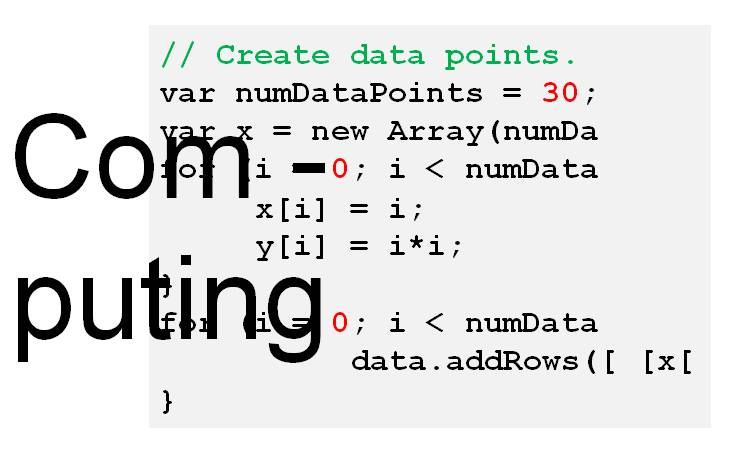# Computing and Computational Analysis: A six-part online course

In an increasingly number-filled world, we need to analyze data with more than a simple calculator; systems as diverse as economic fact sheets, the stock market, sports scores, even poker odds. This online course looks at a number of methods to investigate data, starting with the simplicity of BASIC and Excel, and moving on to more comprehensive programming tools such as JavaScript, Java, Matlab and C. Along the way, youll learn the basics of programming as well as the underlying math and, most importantly, how to mine and analyze data.

The course is divided into six sections. In each, Ill give an introduction to a computational concept and you can do the exercises to see how its done. Most of the applications are freely available on the internet, and I will direct you to the appropriate download site. Computing and computational analysis is hands-on, and best learned by doing. The learning curve gets easier as you go. Enjoy!

Part One

Part Two (n.y.a.)

4. More internet programming (subroutines) [Java]

5. Scientific data analysis [Fortran, Matlab]

6. Object-oriented programming [VB and C]

# 1. Elementary concepts (loops, arrays, simple statistics) [GWBasic]

As its name implies, BASIC is basic and provides a simple programming environment to get one started on the computational road. BASIC was included free with the original Microsoft PC DOS, and is still free to use. Want to calculate the average of 10 test scores (including standard deviation) in an easy way? Just enter the data into a BASIC DATA statement (a list of numbers separated by commas) and run a canned program. I know, most of us would probably input the data into a spreadsheet, but spreadsheets arent very programmable. Well use BASIC to get us going to explain the fundamentals of programming, such as loops and arrays, as well as some elementary statistics. After that, we can move on to more sophisticated languages with real computational power.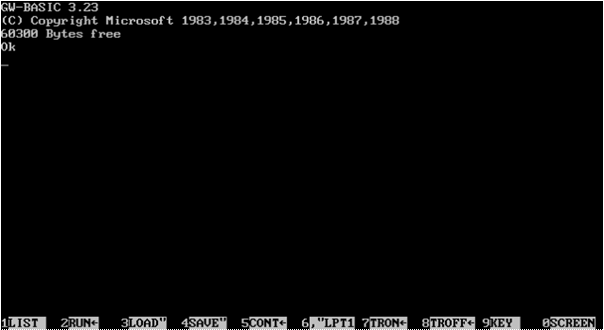Notes:   There are ten function keys, of which well use only the first four: F1 (LIST), F2 (RUN), F3 (LOAD), and F4 (SAVE).   There is no full screen mode.

Program 1.1 Equate: A Simple Square Function

1. Download GWBasic and double-click on the icon to launch the DOS app.

2. Type in the following 3-line program (press the Enter key on each line to store the line).

10 X = 3

20 Y = X^2

30 Print X, Y

3. Run the program by typing RUN and pressing Enter. You should get the result as displayed below.

4. Change the input from X = 3 to X = 4 and run the program again. Just cursor up to line 10, change the 3 to a 4, press Enter to input the line, and cursor back down to the RUN line and press Enter again (the mouse doesnt work in a DOS environment).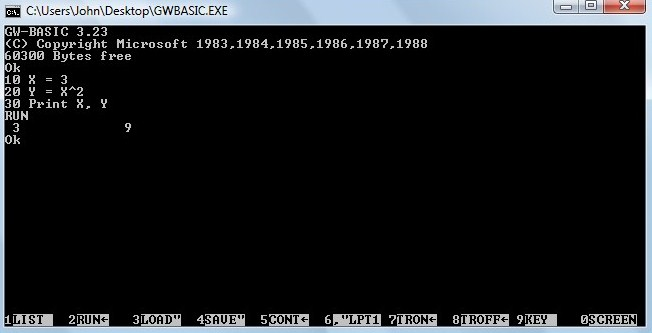Notes:   Every line has a number, which is typically incremented by tens.   y = x2 is coded as Y = X^2 (the syntax of the BASIC power function is ^). Y = X*X is x times x, and gives the same result.   You can press F2 to run the code (saving time as you build and debug a program).

Easy peasy, your first program. Of course, programming wouldnt be very useful if the programs were all as basic as Y = X^2 for X = 3 and X = 4, but the beauty is that the right side of the equation can be as simple as the square function or as complex as working out how to land a lunar module on the moon. Anything really.

Exercise 1.1 How high can you go? In the Equate program, start with X = 2 and increase the exponent until you get an error. What was the value? Why? (Hint: check the original GWBasic screen with message 60300 Bytes free). What form of number was output at Y = 2^24?

Exercise 1.2 What is the square root of 2 to 6 decimal places? Use the Equate program, and recursively bisect X, starting with X = 1.4. What happens when you bisect to the seventh decimal place? Why?

Exercise 1.3 What is the value of the function y = 2x2 - 8x - 3, when x = 4?

Exercise 1.4 What is the value of the function y = 5x4 - 3x3 + 8x2 - 4x + 2, when x = -12?

Program 1.2 Currency converter: Looping or nesting code

The foreign exchange market is a \$5-trillion-a-day business operating almost 24/7. Most trades (83%) such as the USDEUR pair are between the five major currencies: American dollars (USD), euros (EUR), British sterling (GBP), Japanese yen (JPY), and Australian dollars (AUS). As of April 14, 2015, the spot exchange rate of USD to EUR (USDEUR) was 0.9467.

1. Type in the following Currency program and run it. See that you get the same result as shown below. Here, we have looped the conversion function to calculate and print the amount of euros for 1 USD to 10 USD in increments of 1 dollar (using the loop variable I).Notes:   Indenting is good coding practice and makes a program easier to read (and debug).   You could add a print header to make things more readable and print the results to two decimals.

Exercise 1.5 Change the program to include a print header and 2 decimals in the output (PRINT USING "##.##").

Exercise 1.6 Change the program to calculate the conversion output for every half dollar (FOR I = 1 TO 10 STEP .5).

Program 1.3 Stat: Average and standard deviation

1. Input the following Stat program, which calculates the average and standard deviation of 10 numbers (e.g., test scores, bowling averages, weekly food bills). Its a good idea to type the program from scratch if you are just learning to program since Copy and Paste doesnt work in DOS. (You can also Copy and Paste to Notepad and save with a .bas extension.)

The DATA statement lists the marks for 10 scores separated by commas (line 20).

The READ statement stores the marks in the NUMBERS array using a loop (line 30-50). The average is calculated in a sum loop (line 70-90) divided by the number of data points, N (line 130). The standard deviation is calculated in a sum loop (line 100-120) from the sum, the sum of squares, the NUMBERS array, and N (line 140). The results are printed in line 150.

10 N = 10

20 DATA 66,68,72,67,70,66,73,72,70,68

30 FOR I = 1 to N

50 NEXT I

60 SUM = 0: SSQ = 0: AVERAGE = 0: SD = 0

70 FOR I = 1 to N

80   SUM = SUM + NUMBERS(I)

90 NEXT I

100 FOR I = 1 to N

110    SSQ = SSQ + (NUMBERS(I) - SUM/N)^2

120 NEXT I

130 AVERAGE = SUM/N

140 SD = SQR(SSQ/(N-1))

150 PRINT "Ave. = " AVERAGE, "Stn. dev. = " SD

Exercise 1.7 What is the average and standard deviation of the 10 test scores?

Exercise 1.8 Change the program to add 4 scores. What is the average now with the following 4 scores added: 51, 46, 91, 89? Before you run the new data, do you expect the standard deviation to increase, decrease, or stay the same? Why? What is the standard deviation of the 14 scores? Note: All arrays over 10 elements must be dimensioned (DIM). Add the line 11 DIM NUMBERS (N). It will automatically be inserted after line 10 (Type List).

BASIC General Notes

BASIC (Beginners All-purpose Symbolic Instruction Code) began in 1964 at Dartmouth College as a simple high-level language for non-science students, and is partly based on Fortran and Algol. Bill Gates and Paul Allen got their start at Micro-Soft by writing Altair BASIC (1975).

Standard BASIC is interpreted not compiled (runs one line at a time), although executables files (source > object > executable) can be made (e.g., in Quick BASIC). In Quick BASIC (or QBasic) one compiles a BASIC source file to create a BASIC executable or stand-alone .exe run file. Visual BASIC is an object-oriented and event-driven language that is only loosely derived from BASIC.

Everything you wanted to know about BASIC: GW-BASIC User's Guide.

 Pros Cons Special syntax ·  Easy for beginners ·  Quick turnaround for small programs ·  No understanding of computer hardware or operating system ·  Advanced features for experts (string manipulation, graphics, files, data types, subroutines, local variables) · Not very structured · Poor programming practices (e.g., Goto statements) · Quick and easy programs will eventually collapse · Not good output or graphic capability ·    Line numbers required ·    Rem is a comment (remark) ·    : to input multiple statements on one line ·    \$ for text variables ·    For  Next

Note: Windows uses an NT kernel that no longer supports DOS. You can download a DOS-emulated PC Basic here [http://sourceforge.net/projects/pcbasic/postdownload?source=dlp (14 MB)]. Using PC Basic, you can run old BASIC programs in full screen mode with graphics. Here is the SIMPLOT program I wrote to plot data.

# 2. Data mining, correlation, and graphing [Excel]

Excel is not a programming language, but has simple graphing capabilities, easy-to-use statistical functions, and can be used to massage data for use in real programming languages. Excel is a spreadsheet application which displays data and calculations in cells arranged as a grid of rows and columns, e.g., 10 x-y data points in cells A1-B10. Excel is a quick and dirty tool to manipulate data (one cuts and pastes and automatically increments cell arrays in 1-D lists). Apples Viscalc was the first spreadsheet in 1971 and the first killer ap for microcomputers, where the software was more important than the hardware. Lotus and Excel became industry standards, although QuatroPro had good graphing and macro qualities.

Im not going to show you how to use Excel (there are lots of guides out there if needed) other than to highlight how to use the correlation tools, display a R2 correlation coefficient on a graph, and how to write a macro using VBA, Excels programming language.

Exercise 2.1 (Simple graphing). To get started, reproduce the simple y = x2 graph below. (Input cells A2,B2, A3,B3, highlight, and drag to row 12.)

 A B B1 x y (contents) 2 1 1 =A2^2 3 2 4 =A3^2 4 3 9 =A4^2 5 4 16 =A5^2 6 5 25 =A6^2 7 6 36 =A7^2 8 7 49 =A8^2 9 8 64 =A9^2 10 9 81 =A10^2 11 10 100 =A11^2 12 11 121 =A12^2

Exercise 2.2 (Data import). Calculate the average number of homeruns hit per team in the American League and National League for the 2014 MLB season. Which league averaged more? Why? The data can be downloaded here and imported into Excel. See DATA TIP 1 to easily import and massage data. (To calculate the average in the cell below the HR column, use Formulas, Insert Function, and then select Average and OK.)

Exercise 2.3 (Correlation). Plot the number of wins versus team payroll for each MLB team from the 2014 season (Insert | Scatter). What is the relationship between wins and payroll (equation and R2 correlation coefficient)? Display the equation and correlation coefficient on the graph (select the data on the graph, right click, Add Trendline, and tick Display Equation and R-squared). If I had a new team with a payroll of \$130 million, how many wins could I expect? Are sports fair?

(Yes, the data will have to be massaged a bit. Use DATATIP1, sort alphabetically by team, remove the \$ from the payroll data and convert to millions. To convert Excel formulae to data, Paste Special and select Values.)

You should get something like the following graph (without the data labels). Which team got the best bang for its buck? Which team didnt and should probably fire its GM?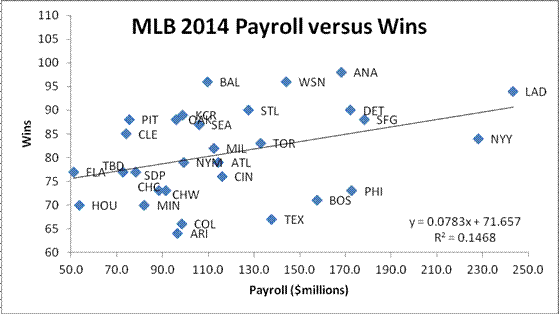Exercise 2.4 (Data Labels). It would be nice to add data labels to see which teams benefit from their high payrolls (or not in the case of the Yankees and others). Since there isnt a built-in Excel label function, we will use VBA (Visual Basic for Applications) to automate the task. You can record any series of tasks in a VBA macro. Here, we add labels on a graph from the cells immediately to the left of the y-x data (Payroll, Wins):

 Team Team Payroll (millions\$) Wins Arizona Diamondbacks ARI 96.6 64 Atlanta Braves ATL 114.5 79 Baltimore Orioles BAL 109.8 96

Adding labels to a graph with a VBA macro:

1. Save the file as an Excel Macro-enabled Workbook.

2. Select Developer | Macros and create a macro called AttachLabelsToPoints.

3. Copy and Paste the subroutine below into the macro window and save.

4. Return to the Excel main view, highlight the graph, and run the macro (Developer | Macros | Run).

Sub AttachLabelsToPoints()

'Dimension variables.

Dim Counter As Integer, ChartName As String, xVals As String

' Disable screen updating while the subroutine is run.

Application.ScreenUpdating = False

'Store the formula for the first series in "xVals".

xVals = ActiveChart.SeriesCollection(1).Formula

'Extract the range for the data from xVals.

xVals = Mid(xVals, InStr(InStr(xVals, ","), xVals, _

Mid(Left(xVals, InStr(xVals, "!") - 1), 9)))

xVals = Left(xVals, InStr(InStr(xVals, "!"), xVals, ",") - 1)

Do While Left(xVals, 1) = ","

xVals = Mid(xVals, 2)

Loop

'Attach a label to each data point in the chart.

For Counter = 1 To Range(xVals).Cells.Count

ActiveChart.SeriesCollection(1).Points(Counter).HasDataLabel = _

True

ActiveChart.SeriesCollection(1).Points(Counter).DataLabel.Text = _

Range(xVals).Cells(Counter, 1).Offset(0, -1).Value

Next Counter

End Sub

Dont worry about how the macro works for now, but note the VBA syntax for a comment (starts with ' and is green), the Do While  Loop, and the For  Next.

Exercise 2.5 (Binomial probability). Download the Random Walk spreadsheet, and play around with it to see the different distributions that result from a drunkard taking 100 steps either right or left out of a pub door (a binomial distribution). To run the simulation, press Enter in Cell I2. (You can also bias the result with a value other than 0.5.) What is the most likely final destination? Why doesnt he end up there after 100 steps? What does it mean: the most likely is not very likely? Is there a symmetry to the distances (i.e., the histogram of data)? Can you explain you answer using the figures below from Chapter 7 in Do The Math!?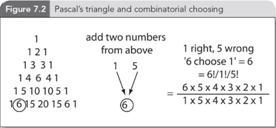The odds of a series of binomial events (coin flips, roulette spins, outcomes of evenly matched playoff series) is elegantly summarized by Pascals triangle. Starting with a 1 for the top row and 1, 2, 1 for the second row, add the two numbers above to get the next line of coefficients, and so on for successive rows.Exercise 2.6 (Calculating binomial odds and Pascals triangle). Pascals triangle can be calculated for any coefficient using n! / r! / (n  r)! / 2n, where n is the row and r is the row coefficient, from 1 to n. For an even 50/50 split, the probability is thus n! / (n/2)! / (n/2!) / 2n. For n = 4, the probability of an even split is 37.5% (4!/2!/2!/24) and for n = 6, 31.25% (6!/3!/3!/26) as shown above in Figure 7.3. What is the chance of an even split for n = 8, 10, and 20? (Note that even splits apply only for even numbers.)

 Pros Cons Special syntax ·  Easy graphing ·  Easy data manipulation and formatting (concatenate with &) ·  Straightforward statistics · Not a programming language, although VBA (Visual Basic for Applications) can create easy-to-use programmed results ·    Equations in a cell begin with = ·    Text begins with  ·    Copy and paste creates new cells ·    Drag and drop expands an arithmetic sequence

DATA TIP 1 Converting online data into useable formats (saves time and ensures data is free from manual transcription errors)

Highlight and copy table (Ctrl+C).

Open Notepad and paste data (Ctrl+V). Save as .txt file (e.g. ALHR2014data.txt). Ignore any Unicode message (OK).

Open Excel, click on Data | From Text and import the .txt file (Choose Finish and OK).

Copy the required column (e.g., HR) and Paste Special | Transpose.

Open Notepad and paste the transposed row (a good trick to get rid of unwanted formatting).

Open Word and paste the data.

Find and replace: tab > , (or to whatever desired data format)

# 3. Internet programming (functions and scripting) [JavaScript]

JavaScript is an interpreted, client-side language. Interpreted  one line at a time (as opposed to a compiled stand-alone executable). Client-side  resides on your machines web browser and doesnt need to access a server. JavaScript gives HTML pages (or scrolls) a bit of oomph, allowing dynamic data manipulation on the fly.

Note that JavaScript is not Java. Java is an object-oriented, platform-independent language that creates stand-alone applets (as well see in Section 4), though the program (and name) is derived from a reduced Java instruction set and the scripting language LiveScript. JavaScript runs programs within a web browser to update a webpage without refreshing, to verify input data in a form, to provide functionality through plugin libraries such as jQuery, and especially to provide unique methods to visualize data. Note, I use Firefox and Windows, but the same principles apply to other browsers (Chrome, Explorer, Safari, Opera) and operating systems.

JavaScript is run within a browser (embedded within the HTML or hyper-text mark-up language code). Hyper-text because we link to other pages and mark-up because text is formatted using tag pairs, for example, to bold a word: <b>Hello</b> produces Hello. Its not WYSIWYG (what you see is what you get) as in a word processor. You have to mark up the text and code with an opening tag and a closing tag, e.g., bold text <b>bold</b> text.

So, lets write our first HTML program.

Use the Notepad++ editor [free download (8MB)] to create the following file. You can Copy and Paste and save as HelloWorld.html. Your default browser icon is automatically associated with the file. Double-click to launch.

<!DOCTYPE HTML>

<html>

<title>Hello World</title>

<body>

<h1>Hello World in HTML (hyper-text mark-up language)!</h1>

This is my first HTML page.

</body>

</html>

Note the opening and closing html, head, and body tags.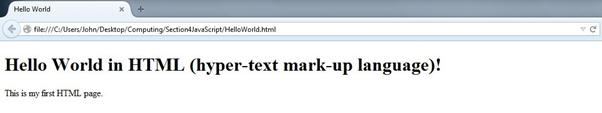Note: If you right-click on the page and select View page source, youll see the source code, a useful way to see whats underneath a web page (thankfully still open source).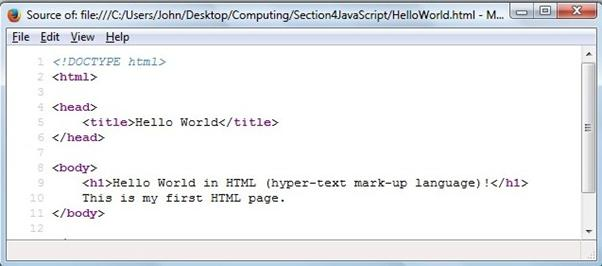Now, lets write our first program with some imbedded JavaScript.

<!DOCTYPE HTML>

<html>

<script>

var message = "Hello World! This is my first JavaScript page."

</script>

<body>

<p>Press the button to display a message.</p>

<button onclick="myFunction()">Press Me</button>

<script>

function myFunction() {

document.write(message);

}

</script>

</body>

</html>

Copy and Paste, save as HelloWorldJavaScript.html, and double-click to launch. Note the opening and closing <script> </script> tags in the head and body text. All JavaScript code is placed between script tags. Note the var statement (variable), the onclick function, and the document.write statement that displays our message. The program isnt very sophisticated, but we have all the basics for writing internet programs that will include analytical and visualization capabilities. And have some fun in the process.

A few beginning notes:

·         JavaScript statements end with ; (JavaScript is a C variant language)

·         Comments begin with // and automatically turn green

·         Functions start with the keyword function and the code is embedded in braces {  }

·         document.write(  ) outputs text and data to the document. It is not recommended, but is used for now as simple static output. Later, well use the document object model (DOM) to change the appearance of a page element (e.g., <h1>, <p>, <div>).

·         Lots of very useful HTML and JavaScript resources are available from w3schools.com: HTML(5) Tutorial and JavaScript Tutorial. A Survey of the JavaScript Programming Language by Douglas Crockford is also excellent.

Exercise 3.1 (Powers of 2). Extend the Powersof2 program to 232 (the relevant body text is shown below). What is 232, 264, and 21000? At what power of 2 is the result = infinity? Why?

<script>

// Calculate and display the powers of two from 2^1 to 2^16.

for (var x = 1, i = 1; i <= 16; i += 1) {

x += x;

document.write('2^' + i, ' = ', x, ' ' + '<br>');

}

</script>

Exercise 3.2 (Pascals Triangle). In Exercise 2.6, we used a visual 100-step Excel Random Walk. We can now calculate the exact odds of a random walker being anywhere after any number of steps (number of reds in a series of roulette spins, heads in a series of coin flips, upticks in a series of stock periods). Use the PascalsTriangle program to determine the chance of being at 0 after n = 8, 10, and 20 steps (the relevant body text is shown below). What are the odds in a best of seven playoff of one team winning 4-0, 4-1, 4-2, and 4-3?

<script>

// Pascal's Triangle.

var N = 16;

var coeffs = [];

// Calculate the rows.

for (var row = 0; row <= N; row += 1) {

coeffs.push([]);

for (var column = 0; column <= row; column += 1) {

if (column === 0 || column === row) {

coeffs[row][column] = 1;

} else {

coeffs[row][column] = coeffs[row-1][column-1]

+ coeffs[row-1][column];

}

}

}

// Display the rows.

for (var row = 1; row < coeffs.length; row += 1) {

document.write("Row " + row + ": "

+ coeffs[row].join(' ') + '<br>');

}

</script>

For some binomial background, check out the article Patterns in Probability: How to See Binomial Statistics (The International Year of Statistics) and the video The Normal Curve (Do The Math! in 100 seconds).

 Binomial (discrete)Gaussian (continuous)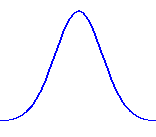Pascals triangle is perfectly suited for a limited numbers of rows, but to determine coefficients for higher rows, we need something more advanced. The Gaussian curve (a continuous version of the discrete binomial function) was discovered for just this reason by French mathematician Abraham de Moivre, who wanted to work out higher-order tiers in Pascals triangle. As you will see in the PascalsTriangle program, we start to run out of memory a little after N = 1000.

Exercise 3.3 (Pascals triangle becomes a Gaussian). What are the odds of exactly breaking even after 1,000 trades? (Screen printing uses a lot of resources, so move the document.write statement out of the loop and print only the last line.) What are the odds of exactly breaking even after 2,000 trades? (You will need to use the Gaussian function to work out the odds of exactly 1,000 H and 1,000 T in 2,000 coin flips.)

A short (Dynamic) HTML aside: The Document Object Model (DOM)

HTML is a tree language, where below the document level (the web page), there are various body elements, such as <h1>, <p>, <div>, etc., which are containers not just separators, the appearance of which can be changed in scripting. For example, I can add a dynamic message to be updated on the fly. The syntax is a bit muddy, but we declare an element in our script and then write to that element as indicated below:

Declare element: document.getElementById("myElement").innerHTML =

Element: <p id="myElement"></p>

Copy and Paste the HelloWorldMessage program, save, and double-click to launch. You can now display HTML pages dynamically, the main reason why JavaScript is used today.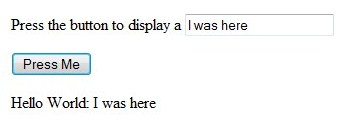Program 3.0 HelloWorldMessage: A dynamic output

<!DOCTYPE html>

<html>

<body>

<p>Press the button to display a <input type="text" id="myText" value="message"></p>

<button onclick="myFunction()">Press Me</button>

<script>

function myFunction() {

var x = document.getElementById("myText").value;

document.getElementById("DynamicContent").innerHTML = 'Hello World: ' + x;

}

</script>

<p id="DynamicContent"></p>

</body>

</html>

Data visualization: A time series plotting routine

Now the fun begins. There are many cool plotting programs to help visualize data in effective ways. All require an HTML/JavaScript code mix with calls to existing JavaScript (or jQuery) libraries. I will use Google Charts to start since it is reasonably straightforward to produce a graph without too much fuss. Once were up and running, you can check out Github for more exotic ideas, but lets get the data side working first before thinking about how to add the looks.

The following plot shows the number of wins for the New York Yankees and the Toronto Blue Jays from 1977-2014 followed by the PlotLine.html program that produced it. Note the <head> </head> section where all the scripting goes and the <body> </body> section with its single <div> element (id = curve_chart) the page container where all the dynamic output goes.Program 3.1 PlotLine: Plot using imbedded data

<html>

<script type="text/javascript"

'modules':[{

'name':'visualization',

'version':'1',

'packages':['corechart']

}]

}"></script>

<script type="text/javascript">

function drawChart() {

['Year', 'Toronto', 'New York'],

['1977',  54,  100],

//

['2014',  83,  84],

]);

var options = {

title: 'Wins per year (1977-2014)',

curveType: 'function',

legend: { position: 'right' }

};

chart.draw(data, options);

}

</script>

<body>

<div id="curve_chart" style="width: 800px; height: 600px"></div>

</body>

</html>

Exercise 3.4 (Plotting time series). Recreate the graph of MLB wins per year using the PlotLine program. (You will need to massage and add the New York and Toronto data.)

Exercise 3.5 (Plotting time series). Plot the U.S., Chinese, and Japanese GDP since 1984, using the PlotLine program. Use the World Bank GDP per capita data (current US\$) and Trading Economics population data.

Exercise 3.6 (Plotting time series). Plot the five quintiles of U.S. household income from 1967 to 2013 using the PlotLine program. Use the U.S. Census Bureau data in Table H-3 (Mean Household Income Received by Each Fifth and Top 5 Percent).

Plotting an equation using PlotFunction.

There are three ways to introduce data into a program: 1) Hard-wired into a data statement (as above in the PlotLine programs), 2) analytically created in code: y = f(x) for a given function, or 3) read from a data file (or database). In the next exercise, we will get data analytically from an equation in a loop and in the following exercise from a file. With these three methods, you can plot anything.

Exercise 3.7 (Plotting a function). We can calculate a data series rather than assigning variables in a long list. The PlotFunction program below shows how to initialize 2 arrays (x and y) and how to populate the array with data from a simple function, y = x2. Change the PlotFunction program below to plot y = 2x2  8x  3. Change the program to plot a sine and a cosine function for 2 periods. Your results should look like those following. (Note the Math.pow function and Math.sin and Math.cos functions.)

Program 3.2 PlotFunction: Plot using equation data

<html>

<script type="text/javascript">

function drawBackgroundColor() {

// Create data points.

var numDataPoints = 30;

var x = new Array(numDataPoints); var y = new Array(numDataPoints);

for (i = 0; i < numDataPoints; i++) {

x[i] = i;

y[i] = i*i; // y = x^2 function

}

for (i = 0; i < numDataPoints; i++) {

}

// Set chart options.

var options = {

title: 'Plotting a function using Google Line Chart', titleFontSize: 20,

hAxis: { title: 'x', titleFontSize: 30, textStyle: {color: '#000', fontSize: 20} },

vAxis: { title: 'y', titleFontSize: 30, textStyle: {color: '#000', fontSize: 20} },

legendFontSize: 20,

lineWidth: 4,

width: 1000,

height: 500

};

// Draw chart.

chart.draw(data, options);

}

</script>

<body>

<div id="chart_div"><div>

</body>

</html>Exercise 3.8 (Plotting from a file). We can also plot data from a file, although for security reasons, reading data locally is typically restricted in the browser sandbox. We can work around this for now, but for security and better functionality data files are best downloaded from a server-side database. Here, we plot data from a file (Dow Jones daily closing value from this year) using the PlotDataFile code (mostly D3 style attributes). Plot the S&P 500 or NASDAQ closing values for the year using the PlotDataFile program and appropriate data file.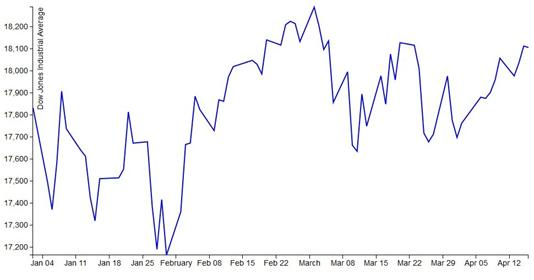So whats the big deal?

Okay, so you might think we have only reinvented an already working wheel, plotting data that is easily plotted in Excel. But the main difference is that we can now do sophisticated analysis on the data before plotting. Or make the data sing using dynamic JavaScript functionality with exotic animations. There are numerous existing programs from the Google Chart Gallery and D3 Gallery. Lets start with the canned Google Chart glitz of a Geo Chart that plots demographic data on a map.

Exercise 3.9 (Geo data). Here is the code and output of a Geo Chart for the U.S. Electoral College. Make a Geo Chart for the world and/or Europe for population, GDP, debt, . (Bonus: select the tool tip attribute from an Option box input.)

<html>

<script type="text/javascript">

function drawRegionsMap() {

['Alabama', 9],

//

['Wisconsin', 10],

['Wyoming', 3]

]);

var options = {'region':'US','resolution':'provinces'};

chart.draw(data, options);

};

</script>

<body>

<div id="chart_div" style="width: 1000px; height: 500px;"></div>

</body>

</html>Exercise 3.10 (Five-card poker hands).

The following two poker hands were generated by the FiveCardPoker program. Use conditional statements (If ... Else) to evaluate which is the winning hand.<!DOCTYPE html>

<body>

Display 2 random 5-card poker hands from a 52-card deck<br>

<script>

// The deck.

// var suit = [ "C", "D", "H", "S" ];

var suit = [ "&clubs;", "&diams;", "&hearts;", "&spades;" ];

var rank = [ "2", "3", "4", "5", "6", "7", "8", "9", "T", "J", "Q", "K", "A" ];

var deck = [];

var N = 52; var H = 5;

// Initialize the cards in the deck.

for (var i = 0; i < rank.length; i++)

{     for (var j = 0; j < suit.length; j++)

{     deck[suit.length*i + j] = suit[j] + rank[i];

}

}

// Shuffle the deck.

var pick; var temp;

for (var i = 0; i < N; i++)

{

pick = Math.floor(Math.random()*N);

temp = deck[pick];

deck[pick] = deck[i];

deck[i] = temp;

}

// Print out the 2 hands.

document.write('Hand 1: '); for (var i = 0; i < H; i++) {document.write(deck[i] + " "); };

document.write('<br>')

document.write('Hand 2: '); for (var i = H; i < 2*H; i++) {document.write(deck[i] + " "); }

</script>

</body>

</html>

 Pros Cons Special syntax ·  Exciting dynamic HTML is the future ·  DOM dynamically changes appearance and behaviour of previously static elements (containers not just seperators) ·  Lots of free canned programs and libraries · Some muddled syntax · Mixes style formatting of CSS and HTML tags · Doesnt do arrays well for numerical analysis · Not the best debugging platform ·    var x = [] is an array ·    Function variables have function scope; without var global scope ·    Dot syntax, e.g., object.property ·    Tag pairs open and close

Cogswell, J. (2014). 5 Programming Languages Youll Need Next Year (and Beyond). Dice. Retrieved from http://news.dice.com/2014/07/29/5-programming-languages-youll-need-next-year-beyond/?icid=ON_DN_UP_JS_AV_OG_RA_2

Guo, P. (2014). Python is Now the Most Popular Introductory Teaching Language at Top U.S. Universities. BLOG@CACM. Retrieved from http://cacm.acm.org/blogs/blog-cacm/176450-python-is-now-the-most-popular-introductory-teaching-language-at-top-us-universities/fulltext

Thackray, A., Brock, D. C., and Jones R. (2015). Fateful Phone Call Spawned Moores Law [Excerpt] Scientific American, April 17, 2015. Retrieved from http://www.scientificamerican.com/article/fateful-phone-call-spawned-moore-s-law-excerpt/?page=1

Web Design (2014). 20 superb data visualisation tools for web designers. Creative Bloq. Retrieved from http://www.creativebloq.com/design-tools/data-visualization-712402

Wissel, L. (1999). How does a logic gate in a microchip work? Scientific American, October 21, 1999. Retrieved from http://www.scientificamerican.com/article/how-does-a-logic-gate-in/

# PROJECTS

Project 1 (Gaussian select). Calculate a z-score with animated graph.

Projecr 2 (Monopoly Monte Carlo). Calculate the odds of landing on any Monopoly square and plot the result.

Project 3 (Blackjack/Poker odds). Calculate the Blackjack odds for hitting/standing against any dealer up card OR calculate the Hold Em poker odds for any head-to-head 2-card matchup.

# Sample Programs

 HTML first program HTML + JavaScript first program Dynamic HTML 2^N, N = 1 to 16 Pascals triangle to 16 rows Plot in-program data Plot a function ( y = x^2) Plot two trig functions Plot Dow Jones data from a file Dow Jones data file 2015 Geo Map U.S. Electoral College votes Deal 2 5-card poker hands• How do pyramid scams work? • Are reality television shows fair? • • Why are sports so uncompetitive? • Who really broke the bank in 2009? • Description Do the Math! is a refreshing look at mathematical concepts and moral dilemmas in the increasingly more numerate world of everyday living. Aiming to popularize and inform, Do the Math! does for numbers what Eats, Shoots, and Leaves does for words. Quotes and comments "One can pop it open anywhere with equal reward." "The style is VERY easy to read and unusually entertaining." "The core concept of the inherent morality of mathematics is excellent."                      Sage      Amazon      Intro      Reviews